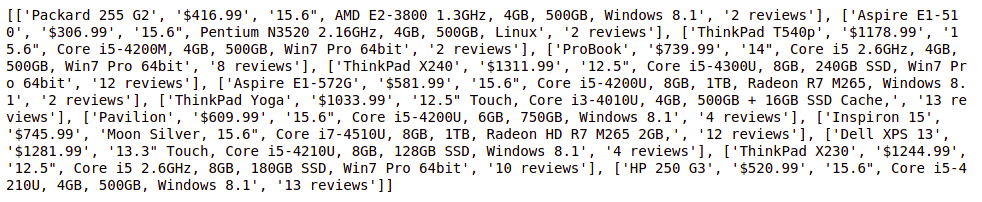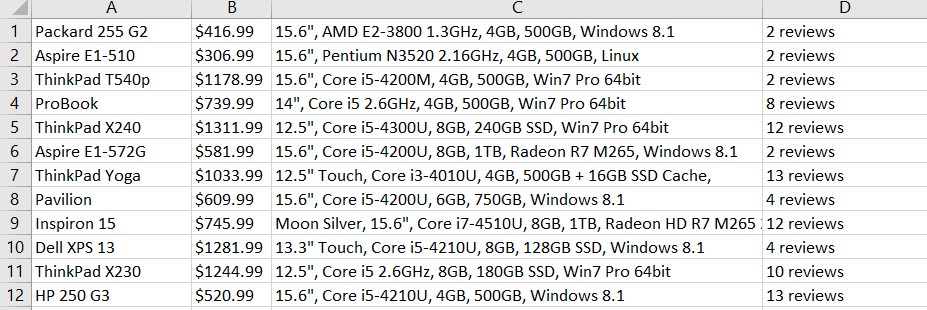GFG App
Open AppBrowser
Continue

# How to scrape multiple pages using Selenium in Python?

As we know, selenium is a web-based automation tool that helps us to automate browsers. Selenium is an Open-Source testing tool which means we can easily download it from the internet and use it. With the help of Selenium, we can also scrap the data from the webpages. Here, In this article, we are going to discuss how to scrap multiple pages using selenium.

There can be many ways for scraping the data from webpages, we will discuss one of them. Looping over the page number is the most simple way for scraping the data. We can use an incrementing counter for changing one page to another page. As many times, our loop will run, the program will scrap the data from webpages.

First Page URL:

`https://webscraper.io/test-sites/e-commerce/static/computers/laptops?page=1`

At last, the Only page numbers will increment like page=1, page=2… Now, Let see for second page URL.

Second Page URL:

`https://webscraper.io/test-sites/e-commerce/static/computers/laptops?page=2`

Now, Let discuss the approach

Installation:

Our first step, before writing a single line of code. We have to install the selenium for using webdriver class. Through which we can instantiate the browsers and get the webpage from the targeted URL.

`pip install selenium`

Once selenium installed successfully. Now, we can go to the next step for installing our next package.

The next package is webdriver_manager, Let install it first,

`pip install webdriver_manager`

Yeah! We are done with the Installation of Important or necessary packages

Now, Let see the implementation below:

• Here in this program, with the help of for loop, We will scrap two webpages because we are running for loop two times only. If we want to scrap more pages, so, we can increase the loop count.
• Store the page URL in a string variable page_url, and increment its page number count using the for loop counter.
• Now, Instantiate the Chrome web browser
• Open the page URL in Chrome browser using driver object
• Now, Scraping data from the webpage using element locators like find_elements method. This method will return a list of types of elements.  We will store all necessary data inside the list variable such as title, price, description, and rating.
• Store all the data as list of list of a single product. In element_list, we will store this resultant list.
• Finally, Print element_list. Then close the driver object.

## Python3

 `# importing necessary packages` `from` `selenium ``import` `webdriver` `from` `selenium.webdriver.common.by ``import` `By` `from` `webdriver_manager.chrome ``import` `ChromeDriverManager`   `# for holding the resultant list` `element_list ``=` `[]`   `for` `page ``in` `range``(``1``, ``3``, ``1``):` `  `  `    ``page_url ``=` `"https://webscraper.io/test-sites/e-commerce/static/computers/laptops?page="` `+` `str``(page)` `    ``driver ``=` `webdriver.Chrome(ChromeDriverManager().install())` `    ``driver.get(page_url)` `    ``title ``=` `driver.find_elements(By.CLASS_NAME, ``"title"``)` `    ``price ``=` `driver.find_elements(By.CLASS_NAME, ``"price"``)` `    ``description ``=` `driver.find_elements(By.CLASS_NAME, ``"description"``)` `    ``rating ``=` `driver.find_elements(By.CLASS_NAME, ``"ratings"``)`   `    ``for` `i ``in` `range``(``len``(title)):` `        ``element_list.append([title[i].text, price[i].text, description[i].text, rating[i].text])`   `print``(element_list)`   `#closing the driver` `driver.close()`

Output:Storing data in Excel File:

Now, We will store the data from element_list to Excel file using xlsxwriter package. So, First, we have to install this xlsxwriter package.

`pip install xlsxwriter`

Once’s installation get done. Let’s see the simple code through which we can convert the list of elements into an Excel file.

## Python3

 `with xlsxwriter.Workbook(``'result.xlsx'``) as workbook:` `    ``worksheet ``=` `workbook.add_worksheet()`   `    ``for` `row_num, data ``in` `enumerate``(element_list):` `        ``worksheet.write_row(row_num, ``0``, data)`

First, we are creating a workbook named result.xlsx. After that, We will consider the list of a single product as a single row. Enumerate the list as a row and its data as columns inside the Excel file which is starting as a row number 0 and column number 0.

Now, Let’s see its implementation:

## Python3

 `import` `xlsxwriter` `from` `selenium ``import` `webdriver` `from` `selenium.webdriver.common.by ``import` `By` `from` `webdriver_manager.chrome ``import` `ChromeDriverManager`   `element_list ``=` `[]`   `for` `page ``in` `range``(``1``, ``3``, ``1``):` `  `  `    ``page_url ``=` `"https://webscraper.io/test-sites/e-commerce/static/computers/laptops?page="` `+` `str``(page)` `    ``driver ``=` `webdriver.Chrome(ChromeDriverManager().install())` `    ``driver.get(page_url)` `    ``title ``=` `driver.find_elements(By.CLASS_NAME, ``"title"``)` `    ``price ``=` `driver.find_elements(By.CLASS_NAME, ``"price"``)` `    ``description ``=` `driver.find_elements(By.CLASS_NAME, ``"description"``)` `    ``rating ``=` `driver.find_elements(By.CLASS_NAME, ``"ratings"``)`   `    ``for` `i ``in` `range``(``len``(title)):` `        ``element_list.append([title[i].text, price[i].text, description[i].text, rating[i].text])`   `with xlsxwriter.Workbook(``'result.xlsx'``) as workbook:` `    ``worksheet ``=` `workbook.add_worksheet()`   `    ``for` `row_num, data ``in` `enumerate``(element_list):` `        ``worksheet.write_row(row_num, ``0``, data)`   `driver.close()`

Output:Output file.